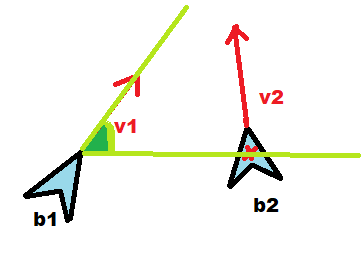# Result of subtracton vectors

Hello, lets asume I have got 2 boids (/agents/objects/whatever) and they are moving forward with some vector v.

[172802-beztytułu.png|172802]

I am wondering what vector is result of that operation ( IN 3D !! ):

Vector3 vector = neighbour.Position -
Position;

And also what angle will it return when compairing these:

float angle =
Vector3.Angle(vector,transform.up);

I wanted to get this green angle - between forward vector v1 and position of v2.
And i am wondering is this right

I would be grateful for answerneighbour.Position - Position is the vector from Position to neighbour.Position. If you start at Position and move by this vector then you end up at neighbour.Position. If you think of the vector as an arrow and place its starting point at Position then the tip will be at neighbour.position.

Using the names from the pictures the green angle can be calculated like this:

`Vector3.Angle(b2.position - b1.position, v1)`

Whether that is the same as what you wrote depends on how those names translate to the names you use in your code.
If b1 is at Position, b2 is at neighbour.Position and transform.up is v1 then it should be correct.